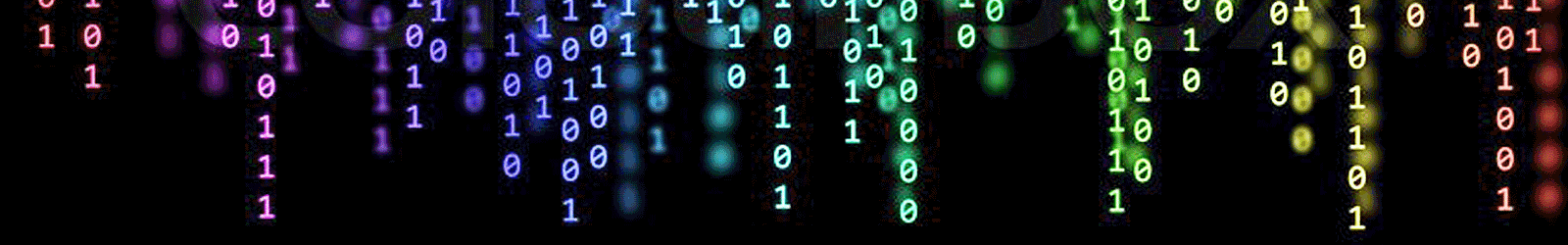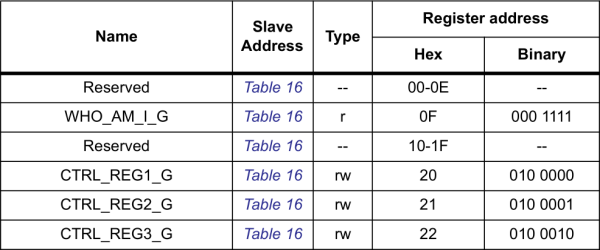# Home » Converting To/From Binary# Converting To/From Binary

Breathe easy! We’ve gotten the hard conversions out of the way. We use hex in electrical and computer engineering because it’s incredibly easy to convert to and from binary – the 1’s and 0’s language of computers.

Converting between hex and binary is easy, because each digit of a hexadecimal number “maps” to four bits (a bit being an individual binary digit) of a binary value. So a byte – eight binary digits – can always be represented by two hexadecimal digits. This makes hex a really great, concise way to represent a byte or group of bytes.

### Converting from Binary to Hex

Let’s begin by mapping the first 16 hexadecimal values to binary.

Decimal Hex Binary Decimal Hex Binary
00 0 0000 08 8 1000
01 1 0001 09 9 1001
02 2 0010 10 A 1010
03 3 0011 11 B 1011
04 4 0100 12 C 1100
05 5 0101 13 D 1101
06 6 0110 14 E 1110
07 7 0111 15 F 1111

As you grow, and continue to use hex and binary, these 16 values will become ingrained in your brain. Those are the key to converting between hex and binary.

To convert between binary and hex, we want to take advantage of the fact that four binary digits (bits) map to one hex digit. Follow these steps to convert from binary to hex.

1. Split a binary value into groups of four, starting at the right-most side.
2. For each group of four, consult the table above to find the matching hex value, and replace groups of four binary digits with the one hex value.

That’s it! Let’s try it out.

#### Binary to Hex Example: Convert 0b101111010100001

To begin, start at the far-right of the binary number, and sort the 1’s and 0’s into groups of four:

 Binary Digits Sorted: 0101 1110 1010 0001

Now consult our big table-o’-sixteen to convert the groups-of-four to a hex digit:

 Binary Digits Sorted: Hex Equivalents: 0101 1110 1010 0001 5 E A 1

And there you have it! 0b101111010100001 = 0x5EA1. (Ugh. This tutorial has far exceeded everyone’s tolerance for 1337. My apologies.)

### Converting from Hex to Binary

Converting from hex to binary is a lot like converting binary to hex. Simply take a hex digit and turn it into four binary digits. Repeat until your number is full of 0’s and 1’s.

#### Hex to Binary Example: Convert 0xDEADBEEF

To convert 0xDEADBEEF (a commonly used code to indicate a system crash), begin by sorting the digits into “bins”:

 Hex Digits Sorted: D E A D B E E F

Then convert each hex digit into four bits:

 Hex Digits Sorted: Hex Digits Sorted: D E A D B E E F 1101 1110 1010 1101 1011 1110 1110 1111

In other words, 0xDEADBEEF = 0b11011110101011011011111011101111. That’s a lot of 1’s and 0’s.

### Use Hex to Represent and Identify Bytes

The examples above show off one of hex’s greatest powers: easily representing values of bytes. Hex is often easier for us to work with because the values are shorter and more memorable than a long string of 1’s and 0’s.